# Three LCOE Methods and Seven Value Drivers

This webpage describes computation of the Levelized cost of electric energy (LCOE) using different methods.  The discussion on this page demonstrates how LCOE for renewable projects can be computed from only four factors for solar power (Cost/kW, capacity factor, fixed O&M and carrying charge rate). For thermal dispatchable plants, the LCOE can be computed from eight factors that include variable O&M, heat rate, fuel cost and availability factor). I have tried to design the page so that you can compute the levelized cost of electricity yourself through understanding the operating and financing cost (i.e. without relying ony on reports that have very colourful football field graphs.)  In addressing LOCE issues, I explain that there are three alternative ways to compute LCOE.  The first method is using the few drivers along with the difficult part, which is to compute carrying charges level carrying charges; the second method is using the formula LCOE = NPV(Revenues)/NPV(Generation); and, the third is computing [FV(Capital Cost) + PV(O&M Cost)]/NPV(Generation).
.
The three alternative LCOE calculations are discussed below beginning with the four/seven factor approach that applies a level carrying charge rate.  The discussion on this page is a bit long and maybe you will say TLNR (too long, not read).  Sometimes I think it is better to explain things in full so you can get to the bottom of the issue. One idea of the page is that LCOE is not a good way to make comparison of intermittent renewable facilities with dispatchable plants.  But you can do various things to get around this problem.  The slides that are attached to the button below contains a power point file where I have tried to put together various ideas in the slides below.
.
.

## Solar Power LCOE Equation from Method 1 – Using Value Drivers and Carrying Charge Rates

For solar, there are four factors that you need to compute LCOE using this first method that include the list below. Please always include these factors in the summary of your financial models:

• Capital Cost/kW
• Fixed O&M Cost/kW-year
• Capacity Factor or Yield (% or kWh/kWp or hours)
• Carrying Charge Rate (Annual Charge divided by Total Cost)

In the case of solar power, the capital cost per kW, the operation and maintenance cost per kW-year and the capacity factor are discussed in the solar resource analysis page. The tricky thing and often the most important factor is the carrying charge rate.  The carrying charge rate is discussed in detail in subsequent pages.  All you have to do is to understand a few formulas.  To see how the formulas are used, make a very crazy assumption that the interest rate and required IRR by investors is zero.  In this case can compute the level cost or the unit cost of solar as using the following steps.

Assume that the solar cost is USD 500/kW and the lifetime of the solar panels is 30 years; the solar O&M is USD 10/kW-year and the capacity factor is 20%.  Without any return on capital (only return of capital), the 500 can be divided by 30 yielding 16.67 per kW-year.  This is like multiplying the 500 by the carrying charge rate — in this case the 30 years is 3.33%.

Capital cost per kW-year = capital cost/kW x carrying charge rate or 500 x 3.33% = 16.67

Next, the capital cost can be converted to an MWH basis by dividing by the hours of operation in a year — conversion of a year to an hour.  To do this you should know that there are 8,760 hours in a non-leap year and that you can convert an MW to a KW through dividing the MW by 1,000. You can take the 16.67 and multiply it by 1000 to derive USD per MW.  Then you can divide this number by 8,760 multiplied by the capacity factor.

Capital cost per MWH = Capital Cost per kW year x 1000/(8760 x capacity factor), or

Capital cost per MWH = 16,667/(8760 x 20%) = 16,667/1,752 = 9.51 USD/MWH

Once the capital cost is derived, the O&M cost can be added.  As the O&M cost is expressed in USD/kW-year, it can be converted to USD/MWH through multiplying by 1,000 and then dividing by the hours operated (8760 x .2) or,

Operating Cost/MWH = Operating Cost/kW-year x 1,000/(8760 x capacity factor), or

Operating Cost/MWH = 10 * 1000/1,752 = 10,000/1,752 = 5.71 MWH

With these two factors, you can add the two components and derive a LCOE of 15.22 per MWH.  These simple equations demonstrate that LCOE can be a pretty easy calculation if the carrying charge is known.

In the exercise file above, you should see how the carrying charge is the EBITDA divided by the investment cost.  For the first case, use the scenario with no inflation.  In this case a flat carrying charge is what the word levelised comes from:  Flat = Level.  The second file below is a completed exercise.

## Illustration of Solar Analysis with Four Factors

The two files below include an exercise where you can work through these equations using a very simple PMT function. You can work through this example in the file to be downloaded below.  The first file has blanks that you can fill-in.  The second file has completed data that you can use to verify the results.

.

To make a LCOE analysis, all you have to do is set-up a little table where you input the four factors.  The excerpts below demonstrate how the drivers of LCOE for solar and demonstrate how to compute LCOE on a nominal basis and a real inflation adjusted basis.  The first excerpt demonstrates the three factors other than the carrying charge rate.The trickiest factor to explain is the carrying charge that converts an one-time cost into an annual cost and recovers return of capital, return on capital (depreciation), taxes, decommissioning costs and other items.  The carrying charge should be computed so that the real on nominal rates are the same over the life of the plant.  Hence the name level.  An illustration of how to compute carrying charge using the PMT function is shown below.  The real rate is computed as (1+nominal cost of capital)/(1+inflation) -1.  Project finance is really all about computing the carrying charge rate.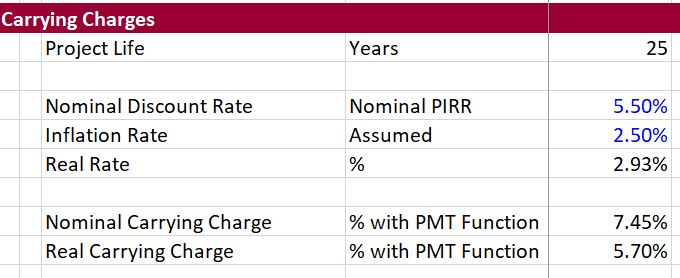Once all of the factors are defined and the carrying charge is established, you can compute the levelised cost of electricity.  You convert the fixed cost per kW-year to the cost per MWH.  Finally, the LCOE can be computed per the formulas above.  For the solar project, the LCOE comes all from fixed costs as illustrated below.I have made many files that evaluate the LCOE for different technologies and included sensitivity analysis.  You can download a couple of these files by pressing on the buttons below.  The screenshot illustrates one of the scenario analyses. A few files that demonstrate how to compute the LCOE.  This file has a sensitivity analysis for the LCOE as illustrated in the screenshot below.
.
.
.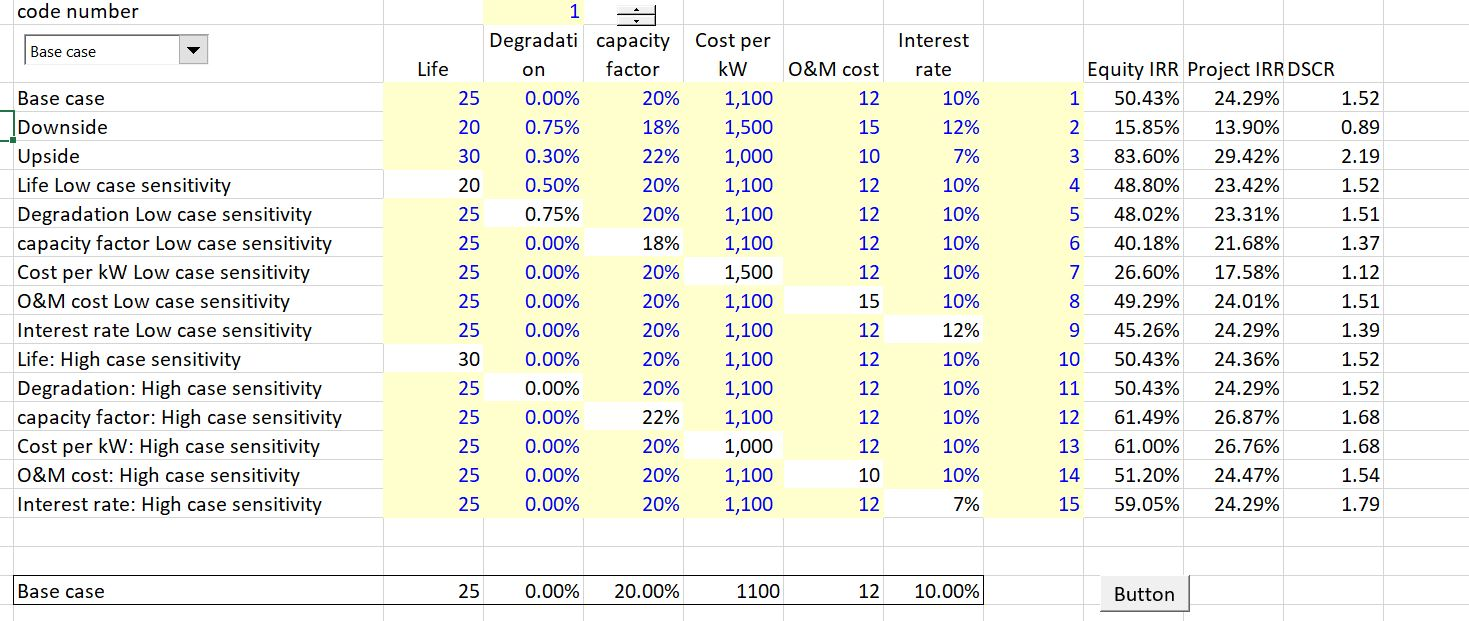.

## LCOE Method 2: – LCOE Formula from NPV Revenue/NPV Cost

The second method for computing LCOE which should give you the same answer as the first method is computing the NPV of revenues at the overall cost of capital and then dividing that number by the NPV of Generation.  This is like taking the NPV of the price.  Many people ask why in the heck would you use the NPV of a physical quantity like MWH in the denominator.  The answer is simply weighting.  If the price is not the same in each period, this approach as the effect of weighting each price by both the present value factor and the relative amount of generation in a period.

In sum, this method which is often used to evaluate different bids by off-takers involves the following formula.

LCOE = NPV(Revenue)/NPV(Generation)

The spreadsheet file below and the associated video prove how this method really is the weighted average price and why it produces a reasonable number.  This method can be illustrated by creating a very simple model with energy generation, revenues the inflate, operating cost and capital expenditures.  Once revenues and generation are computed, the NPV of revenues and generation can be computed. The screen shot below illuatrates the process from LCOE method 2 for three methods.  There are three bids with different price patterns.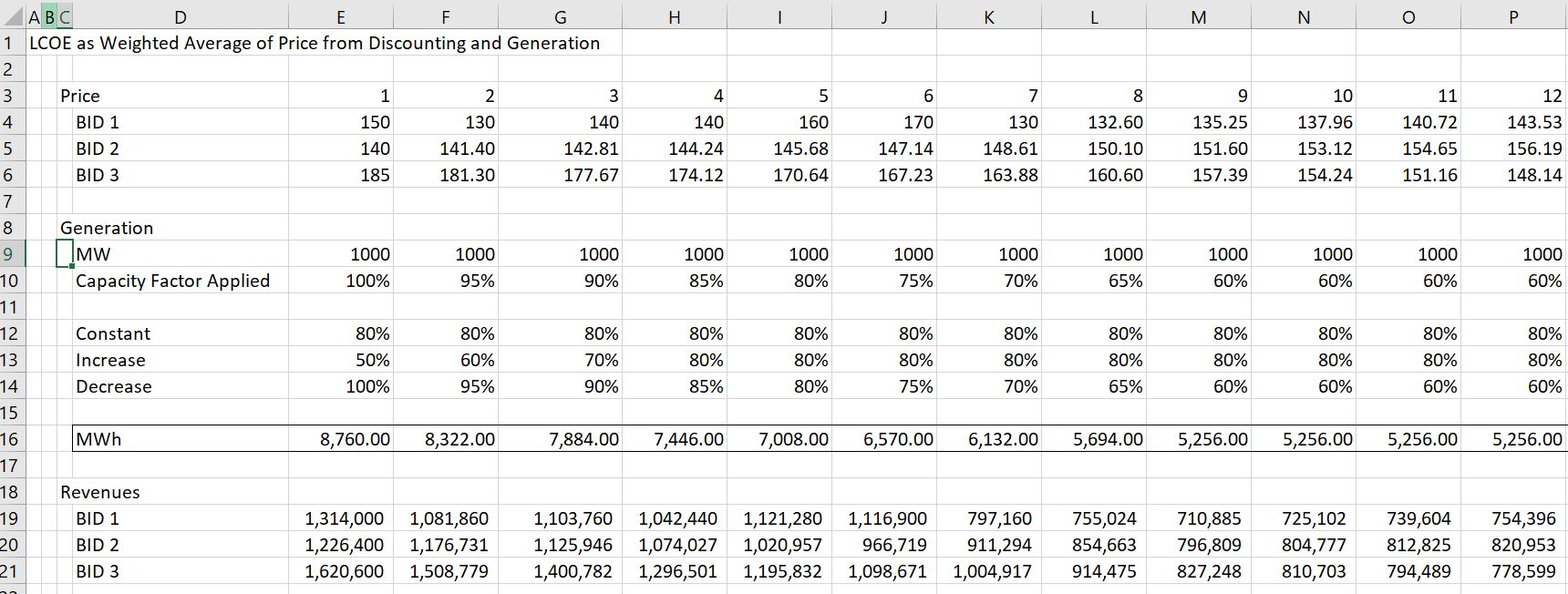To compute LCOE you should account for generation and the PV factor.  You could compute the weighted average of the NPV of generation or you could use the formula.  The equivalence of these methods is demonstrated in the screen shot below.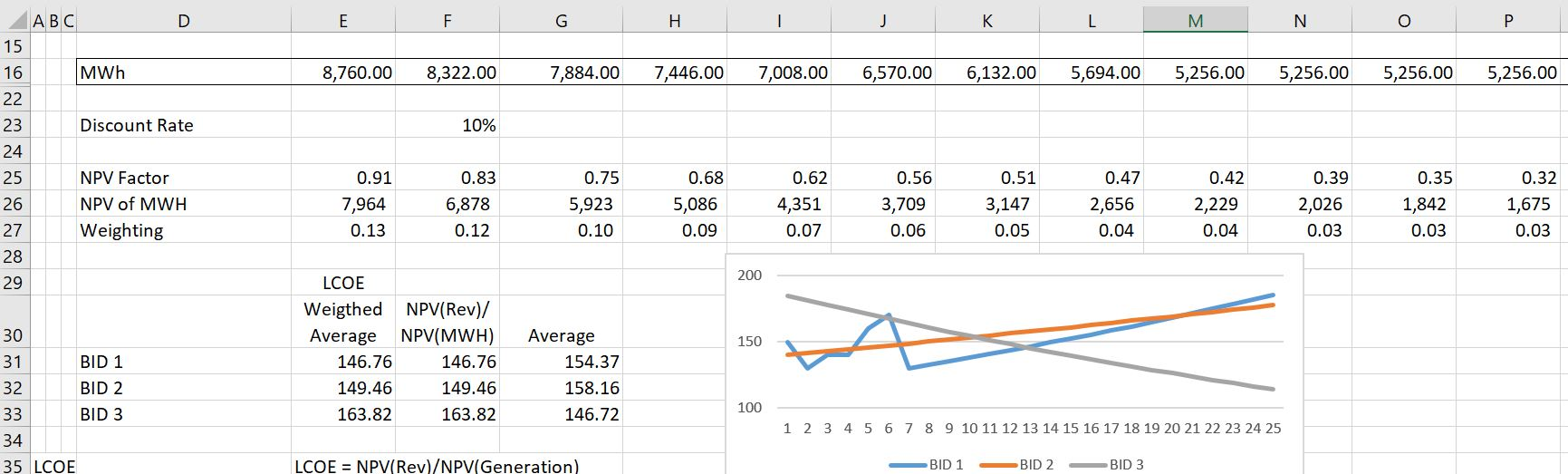With revenues and generation, the LCOE can be computed with the NPV formula below. The first NPV formula reconciles to the LCOE formula above.  The second formula shows that the LCOE process can be used to compute the level O&M per kW-year when the denominator is the capacity rather than energy.

The final calculation is made with the real discount rather than the nominal discount rate.  In this case, the LCOE reconciles to the real LCOE.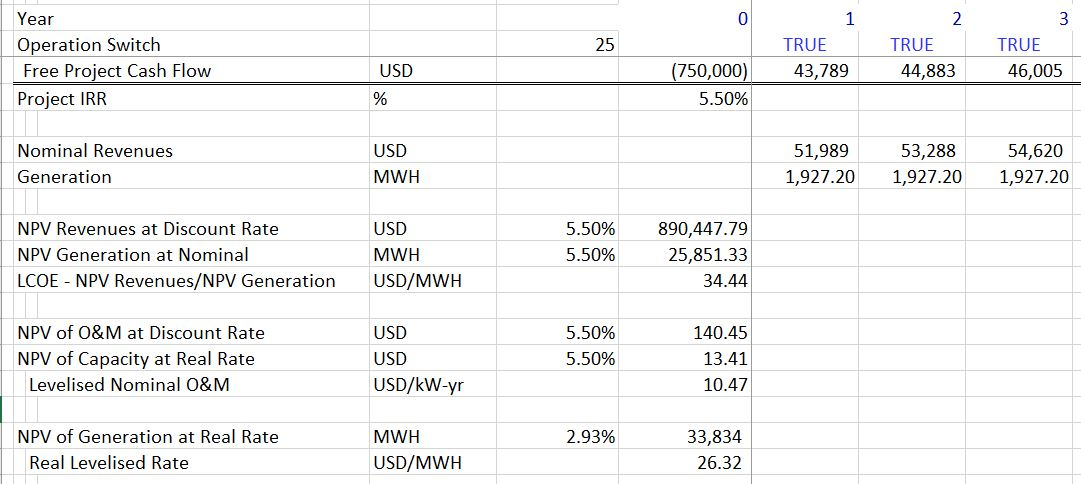## LCOE Method 3 – [Capital Expenditure + NPV(Operating Cost) ]/ NPV (Generation)

As with the first basic LCOE exercise, you can find the exercise to reconcile the financial model with the carrying charge LCOE Method 3: – (Captial Cost + NPV of O&M) Divided by NPV of Generation. The screen shot below shows that the cost method reconciles with the NPV of revenue method.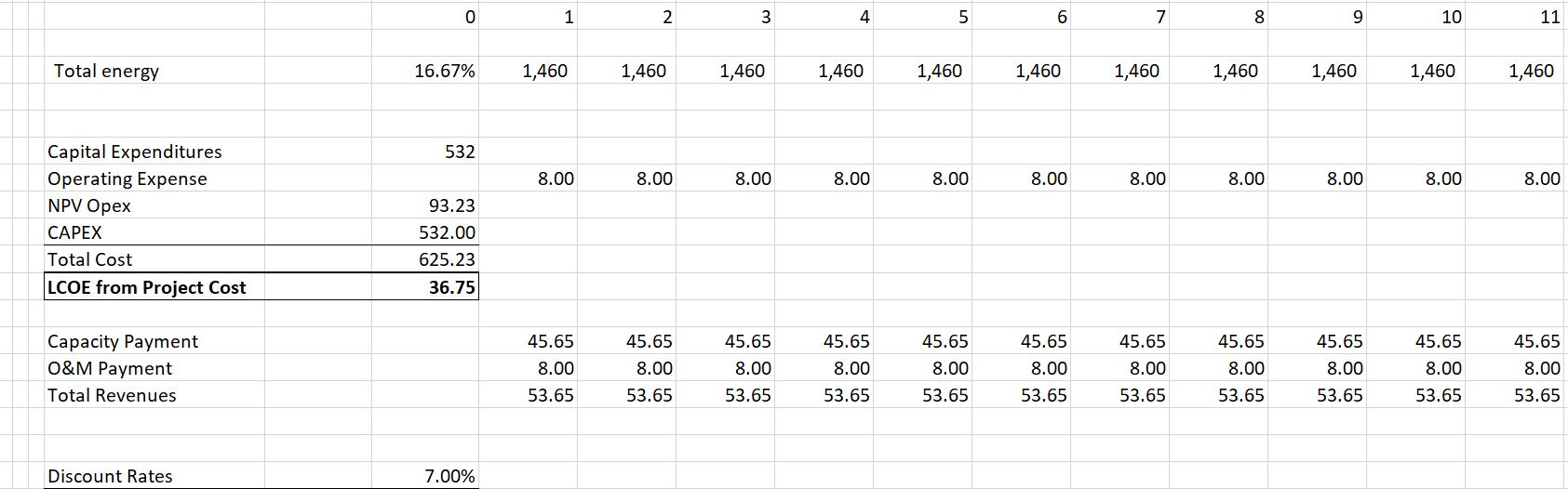This method is described in the videos and the files below (some of them have bad background noise, sorry about that). I then explain that this formula is is the same as the formula for total cost where:

Total Cost = PV of O&M + PV of Capital Expenditure. In this case LCOE = Total Cost/NPV(Generation)

I demonstrate that this formula works only when the discount rate is equal to the PRE-TAX PROJECT IRR.

.

I have made a few other analyses and videos about LCOE.  The video that is attached below addresses how the LCOE for solar can be compared to the variable operating cost of thermal plants so that you do not have to worry about the non-dispatchibility of the solar.  The video also addresses how to compare base load plants with different availability, where you should evaluate the cost of the unavailability of the plant relative to the marginal cost of running other plants during the outage and how this can be incorporated into LCOE.

.

The second video below addresses how to compute the real LCOE in today’s Euro, CFA, GBP, USD etc., rather than the LCOE that is on a nominal basis unadjusted for inflation.  The real LCOE provides a much better measure of current costs and a better comparison of fuel intensive technologies with capital intensive technologies.  The real LCOE can be computed as NPV(Nominal Rate, Nominal Revenues)/NPV(real rate, MWH Generation).  The proof of the real LCOE is shown in the video below.

A third LCOE video describes an LCOE database that I have created which tabulates electricity costs and performance statistics from well known sources including the IEA, Lazard and the EIA.  The video explains how to use the carrying charge spreadsheet along with a database that collects the costs.  The LCOE database is available for download in the file below.  In this file you can compare capital costs, O&M costs, heat rates, renewable capacity factors and other statistics and also evaluate the LCOE.  The video also gives you a little introduction to Power BI.

.

## Equations and Notes for Solar Power LCOE

Given the solar factors  that include (1) capital cost/kW (over night); (2) Fixed O&M cost per kW-year; (3) Capacity Factor Percent or Yield in terms of kWh/kWp; (4) A Carrying Charge Rate that converts capital costs into EBITDA, the steps to compute LCOE are:

• Convert the Cost per kW from an one-time cost to an annual cost. This produces the cost per kW-year. The carrying charge can be thought of as the amount of annual EBITDA required for to support an amount of up-front cost and provide debt investors their return, equity investors their return and pay taxes. You can think of it as like the loan payment on a house divided by the price of the house.

Annual Carrying Charges  (Euro/kW-year) = Cost/kW x Carrying Charge Rate

• Add the fixed O&M costs expressed in Amount (e.g. Euro/kW-year) to the annual carrying charges stated in Euro-kW0year to derive the total annual cost per kW-year.

Total Annual Fixed Cost/kW-year = Annual Carrying Charges + Fixed O&M Cost

• Compute the total fixed cost per unit of energy used rather than capacity. To do this, you need the capacity factor or the yield. The hours that must be covered by the fixed cost are 8766 x capacity factor (8766 instead of 8760 because of leap years). The total annual fixed cost per MWH as the annual cost x 1000 divided by 8766 x capacity factor.

Total Annual Fixed Cost/MWH = Total Fixed Cost/kW-year x 1000/ (8766 x CF)

• Now you are finished — that’s it.  there are no variable costs or fuel costs, so the fixed cost spread over the lifetime of the project on a level basis is the total cost per year divided by the energy provided.

Levelised Cost/MWH = Total Fixed Cost/MWH

Notes for Solar:

1. For economic purposes and when evaluating battery technologies, the cost per MWH should be compared only to the cost/MWH of selling energy.  For example, the cost of a diesel plant.  This is because the capacity of the diesel plant will be needed for times in which the solar plant cannot produce electricity like at night time.
2. For the cost of the solar plant and the diesel to be comparable, the costs must be expressed in real terms or today’s Euro, Ringitts, USD etc. Otherwise you are comparing newborn babies with middle age people.
3. In simple terms, the carrying charge can be computed using the PMT function in excel, where you use the real and not the nominal discount rate.

## LCOE for Dispatchable Plants with Fuel Cost from Method 1 (with Seven Factors)

For dispatchable plants, the costs include the four factors above plus three more for variable O&M and fuel cost as follows:

• Cost/KW
• Fixed O&M Cost/kW-year
• Capacity Factor
• Carrying Charge Rate
• Variable O&M Cost/MWH
• Fuel Price per MMBTU
• Heat Rate: MMBTU/MWH

These drivers are the central issue to any LCOE analysis and include (1) capital cost/kW (over night); (2) Fixed O&M cost per kW-year; (3) Variable O&M cost per MWH; (4) Capacity or availability factor; (5) Heat rate for dispatchable plants (MMBTU/MWH); (6) Fuel cost per MMBTU for dispatchable plants; and (7) Carrying Charge rate that converts capital costs into EBITDA that increases over time.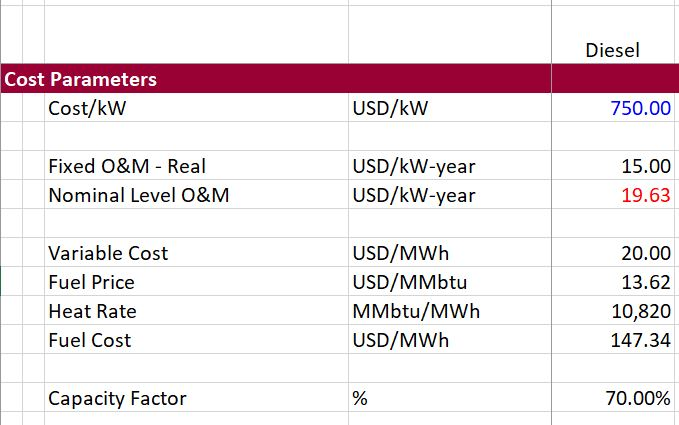The carrying charge rate is the most difficult to work through mechanically. The other factors can be derived from various sources ranging from Lazard LCOE reports to the cost analysis for Chinese coal plants in Pakistan to the using financial models and pvinsights for solar projects.

• Convert the Cost per kW which is the crucial driver of power costs for many technologies by the carrying charge rate. This gives the cost per kW-year. The carrying charge can be thought of as the amount of annual EBITDA required for an amount of up-front cost. It can also be thought of as the return on and the return of capital to carry the investment. It can also be thought of like the loan payment on a house divided by the price of the house.

Annual Carrying Charges = Cost/kW x Carrying Charge Rate

• Add the fixed O&M costs expressed in Amount (e.g. USD) per kW-year to the annual carrying charges to derive the total annual cost per kW-year.

Total Annual Fixed Cost/kW-year = Annual Carrying Charges + Fixed O&M Cost

• Compute the total fixed cost based on energy rather than capacity. To do this, you need the capacity factor (for renewable) or the availability factor for base load (assuming the plant will run whenever it is available). The hours that must be covered by the fixed cost are 8766 x capacity factor. The total annual fixed cost per MWH as the annual cost x 1000 divided by 8766 x capacity factor.

Total Annual Fixed Cost/MWH = Total Fixed Cost/kW-year x 1000/ (8766 x CF)

• Compute the fuel cost as the heat rate expressed in MMBTU/MWH with the Cost (e.g. USD/MMBTU) to derive the fuel cost per MWH.

Fuel Cost (USD/MWH) = Heat Rate (MMBTU/MWH) x Fuel Price (USD/MMBTU)

• Add the variable O&M per MWH to the fuel cost to derive the total variable cost per MWH.

Variable Cost (USD/MWH) = Fuel Cost + Variable O&M

• Add the total fixed cost to the variable cost to derive the levelized cost per MWH.

Levelised Cost/MWH = Total Fixed Cost/MWH + Total Variable Cost/MWH

Notes:

Treat the levelized cost analysis differently for a base load plant and an intermittent low capacity factor renewable energy plant.

For the base load plant, you can measure the effect of different availability by the avoided cost of unavailable time.  You can start with the idea that you want as much energy as possible.  This means you should compute the energy at 100% capacity factor minus the energy that is acutally used.  The difference between the energy for 100% and at the availability factor can be measured at the avoided cost of electricity.

For the intermittent plant with low capacity factor (e.g. solar and low capacity factor wind), measure the levelized cost relative to short-run avoided cost (should include the cost of ancillary services).

For the intermittent plant with a high capacity factor (e.g. off-shore wind and geothermal), could perform a loss of load probability analysis that credits the intermittent plant with some capacity value.

Note that the carrying charge formula is consistent with the formula:

LCOE = NPV(Revenues)/NPV(MWH) when degradation is accounted for in the carrying charges and the carrying charge formula includes inflation. This implies that the carrying charges are computed on a real and not nominal basis if all the fuel and O&M components are inflated on the same basis.

## B.S. in Lazard LCOE Study

Lazard investment bank has published studies on LCOE that have gained a lot of attention.  On television I saw the former govenor of the U.S. state of Michigan refer to the study after somebody well know had made silly statements in a tweet that a cold day disproves the presence of global warming.  The Lazard studies had a whole buch of football field diagrams that may look fancy but don’t really tell you much.  If you want to use the Lazard study you need to know the specific capacity factor and put in a carrying charge rate.  Then you can do something much better and compute the LCOE yourself.  Also, the LCOE should be in real terms not in nomial terms as in the Lazard study.

## Other files in Resource Library

I have not finished uploading all of the LCOE files that have examples of LCOE techniques.  You can get these files by sending me an e-mail at edwardbodmer@gmail.com.  I will eventually upload these files with LCOE comparisons.

• Renewable Project Finance Model with Instructions.xlsm
• Renewable Model with Structuring and Risk Analysis.xlsm
• Renewable Analysis Revised.xlsm
• Renewable Template.xls
• Renewable Screening with Biomass.xlsm
• Renewable Screening Analysis.xlsm